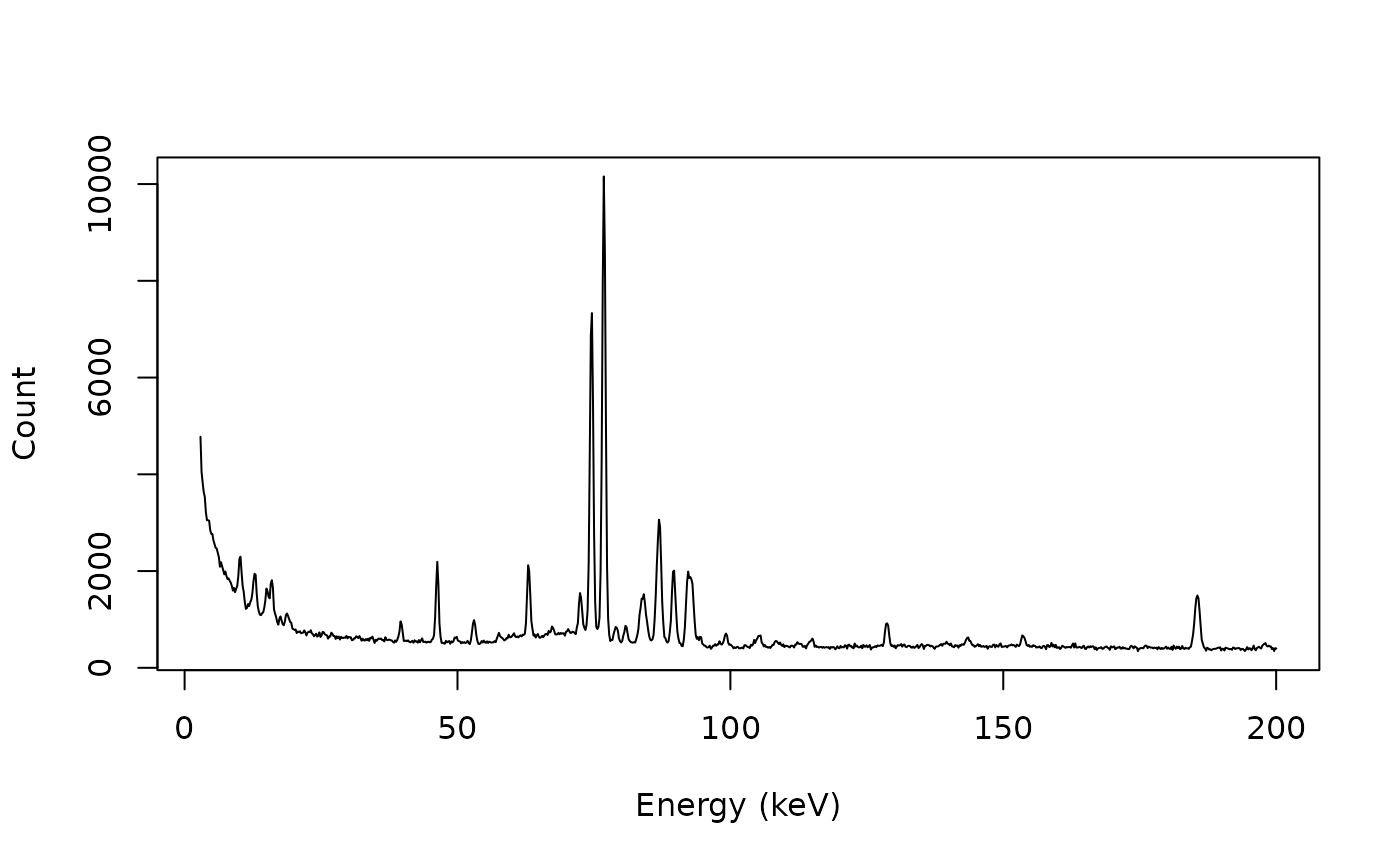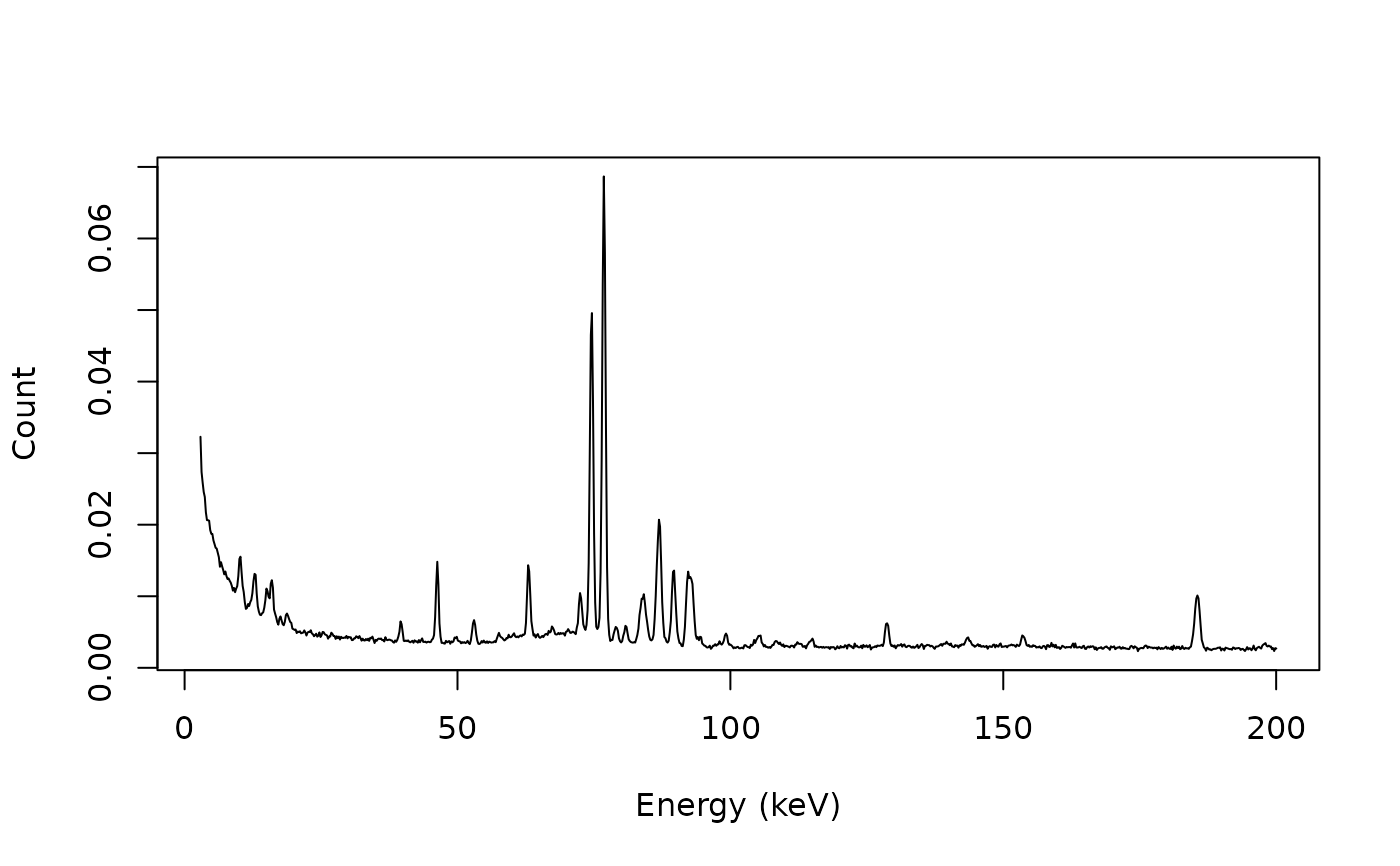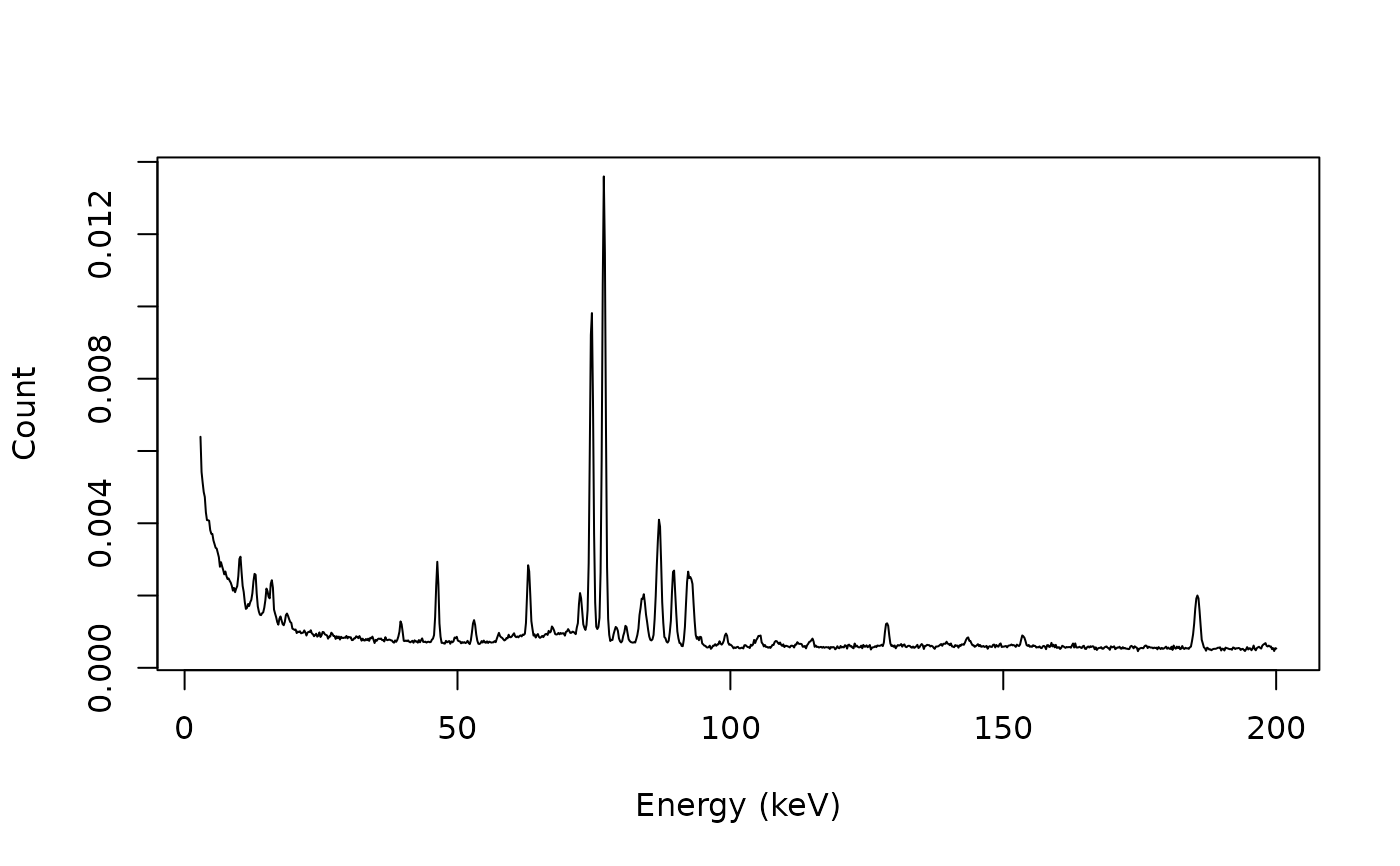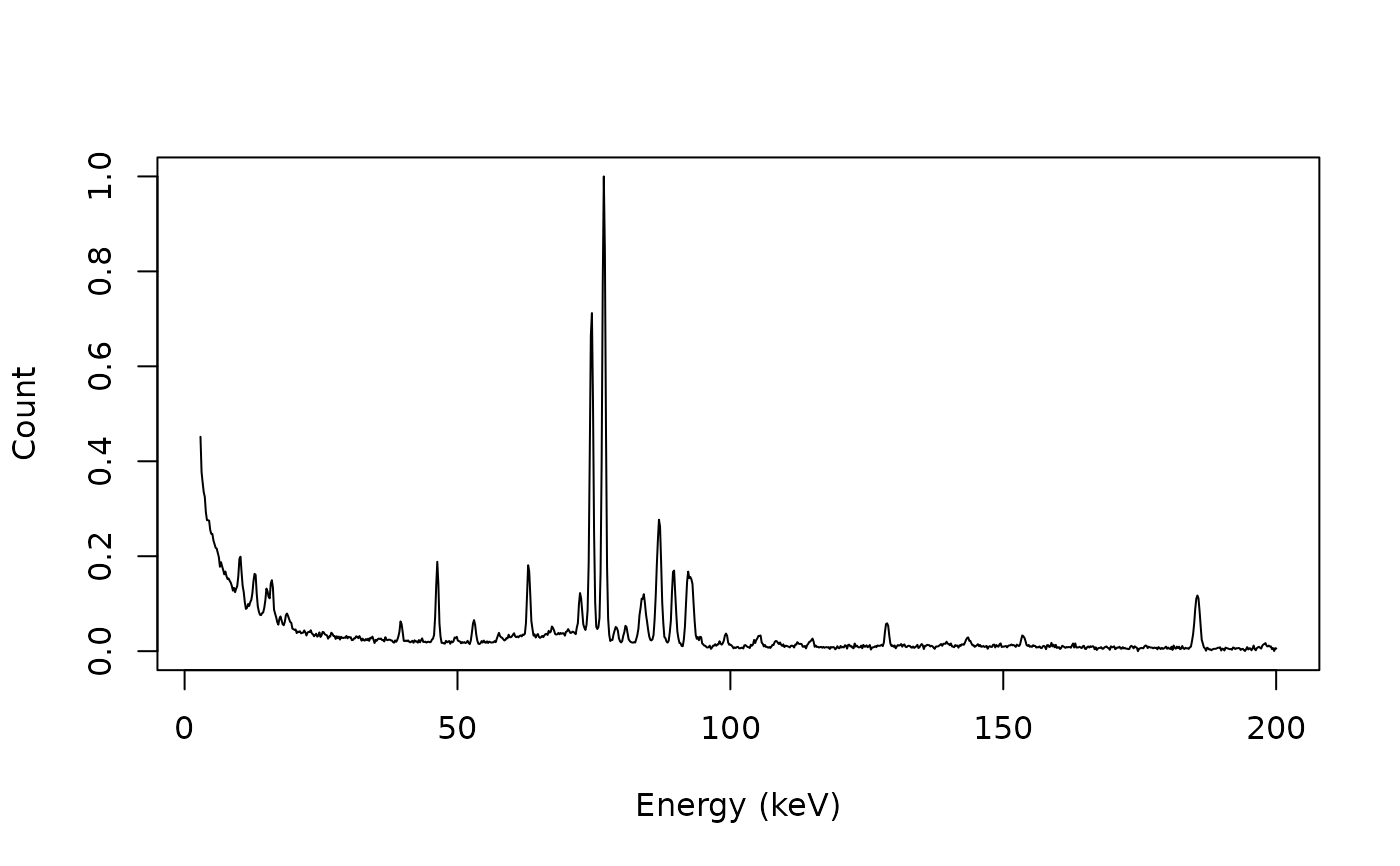Rescales intensities so that the area under the curve (AUC) is equal to 1.

## Usage

rescale_area(x, y, ...)

# S4 method for numeric,numeric
rescale_area(x, y, method = c("rectangle", "trapezoid"), ...)

# S4 method for ANY,missing
rescale_area(x, method = c("rectangle", "trapezoid"), ...)

## Arguments

x, y

A numeric vector. If y is missing, an attempt is made to interpret x in a suitable way (see grDevices::xy.coords()).

...

Currently not used.

method

A character string specifying the method for integration. It must be one of "rectangle" or "trapezoid". Any unambiguous substring can be given.

## Value

Returns a list with two components x and y.

Other normalization methods: rescale_range(), rescale_total(), rescale_transform()

N. Frerebeau

## Examples

## gamma-ray spectrometry
data("BEGe")

## Subset from 2.75 to 200 keV
BEGe <- signal_select(BEGe, from = 3, to = 200)

## Plot spectrum
plot(BEGe, type = "l", xlab = "Energy (keV)", ylab = "Count")## Normalize by area under the curve
BEGe_area <- rescale_area(BEGe)
plot(BEGe_area, type = "l", xlab = "Energy (keV)", ylab = "Count")integrate_rectangle(BEGe)
#>  147922.1
integrate_rectangle(BEGe_area)
#>  1

## Rescale so that intensities sum to 1
BEGe_total <- rescale_total(BEGe, total = 1)
plot(BEGe_total, type = "l", xlab = "Energy (keV)", ylab = "Count")## Rescale intensities to 0-1
BEGe_range <- rescale_range(BEGe, min = 0, max = 1)
plot(BEGe_range, type = "l", xlab = "Energy (keV)", ylab = "Count")